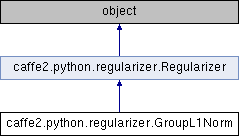Caffe2 - Python API A deep learning, cross platform ML framework
caffe2.python.regularizer.GroupL1Norm Class Reference
Inheritance diagram for caffe2.python.regularizer.GroupL1Norm:## Public Member Functions

def __init__ (self, reg_lambda, groups, stabilizing_val=0)Public Member Functions inherited from caffe2.python.regularizer.Regularizer
def __init__ (self)

def __call__ (self, net, param_init_net, param, grad=None, by=None)

## Public Attributes

reg_lambda

groups

stabilizing_valPublic Attributes inherited from caffe2.python.regularizer.Regularizer
kEpsilon

## Detailed Description

```Scardapane, Simone, et al. "Group sparse regularization for deep neural networks."
Neurocomputing 241 (2017): 81-89.

This regularizer computes l1 norm of a weight matrix based on groups.
There are essentially three stages in the computation:
1. Compute the l2 norm on all the members of each group
2. Scale each l2 norm by the size of each group
3. Compute the l1 norm of the scaled l2 norms
```

Definition at line 237 of file regularizer.py.

## Constructor & Destructor Documentation

 def caffe2.python.regularizer.GroupL1Norm.__init__ ( self, reg_lambda, groups, stabilizing_val = `0` )
```Args:
reg_lambda: The weight of the regularization term.
groups: A list of integers describing the size of each group.
The length of the list is the number of groups.

Optional Args:
stabilizing_val: The computation of GroupL1Norm involves the Sqrt
operator. When values are small, its gradient can be numerically